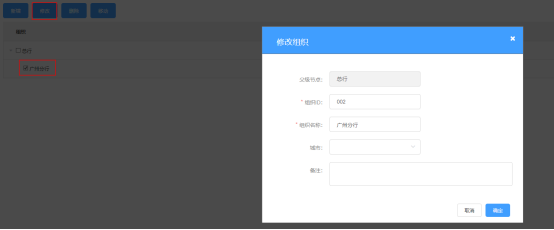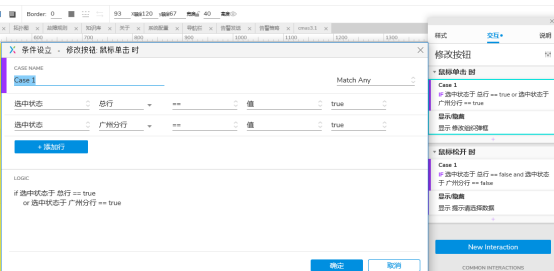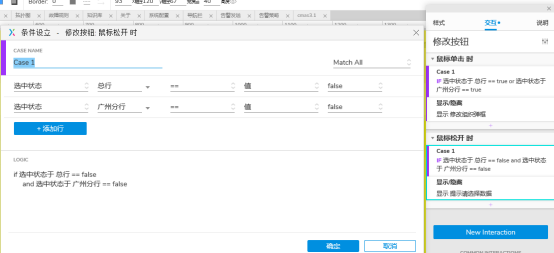• Oracle在where语句后添加日期限制条件
千次阅读
2020-07-24 10:11:35

语法： select t*,t.rowid from DataBaseName where time >= to_date(‘2020-6-1’,‘yyyy-mm-dd’) and time2 <= to_date(‘2020-7-24’,‘yyyy-mm-dd’)

或者是 select t*,t.rowid from DataBaseName where time between to_date(‘2020-6-1’,‘yyyy-mm-dd’) and to_date(‘2020-7-24’,‘yyyy-mm-dd’)oracle
更多相关内容
• 如下图，给"修改"按钮添加 “鼠标松开时-显示影藏” 交互事件，设置为"显示 提示选择数据的弹窗"，触发鼠标松开时事件的条件是"总行"和"广州分行"未被选中 至此配置完成，即可实现当选择框部分一

## 1.需求说明如以上两图，当选择框一个都没选时，点击修改按钮打开的是请选择数据的提示弹窗，当有选择选择按钮时，点击修改按钮打开修改组织弹窗

## 2.实现步骤

1. 如下图，给"修改"按钮添加 “鼠标点击-显示影藏” 交互事件，设置为"显示 修改组织弹窗"，触发鼠标点击事件的条件是"总行"或者"广州分行"被选中2. 如下图，给"修改"按钮添加 “鼠标松开时-显示影藏” 交互事件，设置为"显示 提示选择数据的弹窗"，触发鼠标松开时事件的条件是"总行"和"广州分行"未被选中至此配置完成，即可实现当选择框部分一个都没被选中时，点击修改按钮打开的是"请选择数据的提示弹窗"，当有选中选择按钮时，点击修改按钮打开的是"修改组织弹窗"

展开全文• 从四种基本平差算法的函数模型来看，主要包括如下两种类型的条件方程：{F(L^,X^)=0ϕ(X^)=0\left\{\begin{matrix} F(\hat{L},\hat{X})=0\\ \phi (\hat{X})=0 \end{matrix}\right. 第一种方程中同时含有观测值和...

接下来要写的可看成是对前面讲过的四种经典平差方法的概括模型。
从四种基本平差算法的函数模型来看，主要包括如下两种类型的条件方程： { F ( L ^ , X ^ ) = 0 ϕ ( X ^ ) = 0 \left\{\begin{matrix} F(\hat{L},\hat{X})=0\\ \phi (\hat{X})=0 \end{matrix}\right.
第一种方程中同时含有观测值和未知参数，称为一般条件方程；第二种方程则只含有未知参数而无观测值，称为限制条件方程。
一般而言，对于任何一个平差问题，设想观测值个数为 n n ，必要观测数为 t t ,则多余观测数 r = n + t r=n+t 。若增选了 u u 个参数，不论 u < t , u = t u<t,u=t u > t u>t ,也不论参数是否独立，每增加一个参数则相应的多产生一个方程，故总共应列出 r + u r+u 个方程。如果在 u u 个参数中有 s s 个是不独立的，或者说，在这 u u 个参数之间存在着 s s 个函数关系式，则应列出 s s 个限制条件方程，此外，还应列出 c − r + u − s c-r+u-s 个一般条件方程，如此就形成了如下的函数模型： F c   1 ( L ^ , X ^ ) = 0 ϕ s   1 ( X ^ ) = 0 \begin{matrix} \underset{c\,1 }{F}(\hat{L},\hat{X})=0\\ \underset{s\, 1}{\phi}(\hat{X})=0 \end{matrix} 这就是附有限制条件的条件平差函数模型。其线性形式可概括如下： A c   n V n   1 + B c   u x ^ u   1 + W c   1 = 0 C s   u x ^ u   1 + W x s   1 = 0 \begin{matrix} \underset{c\,n }{A}\underset{n\,1 }{V}+\underset{c\,u }{B}\underset{u\,1}{\hat{x}}+\underset{c\,1}{W}=0\\ \underset{s\,u}{C}\underset{u\,1}{\hat{x}}+\underset{s\,1}{W_{x}}=0 \end{matrix} 式中 W = F ( L , X 0 ) , W x = ϕ ( X 0 ) W=F(L,X^{0}),W_{x}=\phi(X^{0}) 其中， X 0 X^{0} 是未知参数的初始值，一般选择在收敛值的某个开邻域 U U 里，使得 U U 必须和3维欧式空间 R 3 R^{3} 中的一个开子集是同胚的。
c = r + u − s , c > r , s < u c=r+u-s,c>r,s<u ，系数矩阵的秩分别为 R ( A ) = c , R ( B ) = u , R ( C ) = s R(A)=c,R(B)=u,R(C)=s 随机模型为 D n   n = σ 0 2 Q n   n = σ 0 2 P n   n − 1 \underset{n\, n}{D}=\sigma _{0}^{2} \underset{n\, n}{Q}=\sigma _{0}^{2} \underset{n\, n}{P}^{-1} 在大多数情况下，参数之间是等价的，不需要加权，这时候 P P 是单位矩阵。
上面提到过，该模型是四种平差模型的综合：

1. 系数阵中的 B = 0 , C = 0 B=0,C=0 时，它就变成了条件平差法的函数模型。
2. 系数阵中的 C = 0 C=0 ，它就变成了附有参数的条件平差的函数模型。
3. 系数阵中的 A = − I A=-I C = 0 C=0 时，它就变成了间接平差法的函数模型。
4. 系数阵中的 A = − I A=-I 时，就变成了附有限制条件的间接平差法的函数模型。

由此，也称之为“概括平差模型”。
回到正题来简化数学模型，上面的线性模型用拉格朗日乘子进行复合，组成函数如下： ϕ = V T P V − 2 K T ( A V + B x ^ + W ) − 2 K s T ( C x ^ + W x ) \phi=V^{T}PV-2K^{T}(AV+B\hat{x}+W)-2K_{s}^{T}(C\hat{x}+W_{x})
注意，这里有两个拉格朗日乘子，对于拉格朗日乘子，可参看我的另外一篇文章：拉格朗日乘子法的由来
由凸优化理论，要计算该极值，需对 V V x ^ \hat{x} K K K s K_{s} 分别取极值，即是求偏导并令其为零，得 ∂ Φ ∂ V = 2 V T P − 2 K T A = 0 ∂ Φ ∂ x ^ = − 2 K T B − 2 K s T C = 0 A V + B x ^ + W = 0 C x ^ + W x = 0 \begin{matrix} \frac{\partial \Phi }{\partial V}=2V^{T}P-2K^{T}A=0\\ \frac{\partial \Phi }{\partial \hat{x}}=-2K^{T}B-2K_{s}^{T}C=0\\ AV+B\hat{x}+W=0\\ C\hat{x}+W_{x}=0 \end{matrix}
上面四式包含 n n 个改正数， u u 个参数， c c 个对应于一般条件方程的联系数以及 s s 个对应于限制条件方程的联系数，而方程个数为 c + s + n + u c+s+n+u ，即方程个数等于未知数个数，故有唯一解。以上四式成为附有限制条件的条件平差法的基础方程。
解此基础方程，令 N A A = A Q A T N_{AA}=AQA^{T}
N A A K + B x ^ + W = 0 B T K + C T K s = 0 C x ^ + W x = 0 \begin{matrix} N_{AA}K+B\hat{x}+W=0\\ B^{T}K+C^{T}K_{s}=0\\ C\hat{x}+W_{x}=0 \end{matrix}
以上三式称为附有限制条件的条件平差的法方程。
由法方程得 K = − N A A − 1 ( W + B x ^ ) B T N A A − 1 B x ^ − C T K + B T N A A − 1 W = 0 \begin{matrix} K=-N_{AA}^{-1}(W+B\hat{x})\\ \\ B^{T}N_{AA}^{-1}B\hat{x}-C^{T}K+B^{T}N_{AA}^{-1}W=0 \end{matrix} 若令 N B B = B T N A A − 1 B , W e = B T N A A − 1 W N_{BB}=B^{T}N_{AA}^{-1}B,W_{e}=B^{T}N_{AA}^{-1}W 可进一步化简得
N B B x ^ − C T K s + W e = 0 N_{BB}\hat{x}-C^{T}K_{s}+W_{e}=0 解出 x ^ \hat{x} ，得 x ^ = N B B − 1 ( C T K s − W e ) \hat{x}=N_{BB}^{-1}(C^{T}K_{s}-W_{e}) 将上式代入 C x ^ + W x = 0 C\hat{x}+W_{x}=0 ，得 N C C K s − C N B B − 1 W e + W x = 0 N_{CC}K_{s}-CN_{BB}^{-1}W_{e}+W_{x}=0
其中 N C C = C N B B − 1 C T N_{CC}=CN_{BB}^{-1}C^{T} 。解出 K s K_{s} K s = − N C C − 1 ( W x − C N B B − 1 W e ) K_{s}=-N_{CC}^{-1}(W_{x}-CN_{BB}^{-1}W_{e}) 经整理即得： x ^ = − ( N B B − 1 − N B B − 1 C T N C C − 1 C N B B − 1 ) W e − N B B − 1 C T N C C − 1 W x \hat{x}=-(N_{BB}^{-1}-N_{BB}^{-1}C^{T}N_{CC}^{-1}CN_{BB}^{-1})W_{e}-N_{BB}^{-1}C^{T}N_{CC}^{-1}W_{x}
下面是具体的代码实现，其中基本的矩阵运算没有在下面给出，在矩阵算法相关代码，如有需要可以下载。

// <summary>
/// 附有限制条件的条件平差
/// </summary>
/// <param name="correction">返回的改正数</param>
/// <param name="MatrixA"></param>
/// <param name="MatrixB"></param>
/// <param name="MatrixC"></param>
/// <param name="W"></param>
/// <param name="Wx"></param>
/// <param name="C"></param>
/// <param name="N"></param>
/// <param name="U"></param>
/// <param name="S"></param>
//
template<class T>
void GetConditionCorrectionWithCondition(T correction[],const T matrixA[],const T matrixB[],const T matrixC[],const T W[],const T Wx[],int C,int N,int U,int S)
{
T *transposedA=new T[N*C];
T *transposedB=new T[U*C];
T *transposedC=new T[U*S];
T *Naa=new T[C*C];
T *inverseForNaa=new T[C*C];
T *Nbb=new T[U*U];
T *inverseForNbb=new T[U*U];
T *Ncc=new T[S*S];
T *inverseForNcc=new T[S*S];
T *We=new T[U];
T *x=new T[U];
MatrixTranspose(matrixA,transposedA,C,N);
MultMatrix(matrixA,transposedA,Naa,C,N,C);
MatrixAnti(Naa,inverseForNaa,C);
MatrixTranspose(matrixB,transposedB,C,U);
T *transit1=new T[U*C];
MultMatrix(transposedB,inverseForNaa,transit1,U,C,C);
MultMatrix(transit1,matrixB,Nbb,U,C,U);
MatrixAnti(Nbb,inverseForNbb,U);

T *transit2=new T[U*C];
MultMatrix(transposedB,inverseForNaa,transit2,U,C,C);
MultMatrix(transit2,W,We,U,C,1);

T *transit3=new T[S*U];
MultMatrix(matrixC,inverseForNbb,transit3,S,U,U);
MatrixTranspose(matrixC,transposedC,S,U);
MultMatrix(transit3,transposedC,Ncc,S,U,S);
MatrixAnti(Ncc,inverseForNcc,S);

T *transit4=new T[U*S];
MultMatrix(inverseForNbb,transposedC,transit4,U,U,S);
T *transit5=new T[U*S];
MultMatrix(transit4,inverseForNcc,transit5,U,S,S);

T *transit6=new T[U*U];
MultMatrix(transit5,matrixC,transit6,U,S,U);
T *transit7=new T[U*U];
MultMatrix(transit6,inverseForNbb,transit7,U,U);
MatrixMinus(inverseForNbb,transit7,transit7,U,U);
T *transit8=new T[U];
MultMatrix(transit7,We,transit8,U,U,1);

T *transit9=new T[U];
MultMatrix(transit5,Wx,transit9,U,S,1);

MatrixMinus(transit9,transit9,correction,U,1);
MatrixMinus(correction,transit8,correction,U,1);
MatrixMinus(correction,transit9,correction,U,1);

delete [] transposedA;
delete [] transposedB;
delete [] transposedC;
delete [] Naa;
delete [] inverseForNaa;
delete [] Nbb;
delete [] inverseForNbb;
delete [] Ncc;
delete [] inverseForNcc;
delete [] We;
delete [] x;
delete [] transit1;
delete [] transit2;
delete [] transit3;
delete [] transit4;
delete [] transit5;
delete [] transit6;
delete [] transit7;
delete [] transit8;
delete [] transit9;
}


下面进行精度评定，附有限制条件的条件平差的单位权方差估值也是 V T P V V^{T}PV 除以它的自由度，即
σ ^ 0 2 = V T P V r = V T P V c − u + s \hat{\sigma }_{0}^{2}=\frac{V^{T}PV}{r}=\frac{V^{T}PV}{c-u+s}
另外，应用协因数传播率，可得 Q x ^ x ^ = N B B − 1 − N B B − 1 C T N C C − 1 C N B B − 1 Q_{\hat{x}\hat{x}}=N_{BB}^{-1}-N_{BB}^{-1}C^{T}N_{CC}^{-1}CN_{BB}^{-1} 则平差值函数的中误差很容易得到，在这里不想写的太详细了，前面几篇文章详细讨论过。

参考资料：
 误差理论与测量平差基础 武汉大学测绘学院测量平差学科组编著
 变分学讲义 张恭庆著

转载请注明出处：http://blog.csdn.net/fourierFeng/article/details/76862227

展开全文最小二乘 条件平差
• ## sqlserver里面增加约束条件

千次阅读 多人点赞 2018-07-04 19:38:33
1.主键约束：格式为：alter table 表格名称 add constraint 约束名称 增加的... 2.check约束：就是给一列的数据进行了限制格式：alter table 表名称 add constraint 约束名称 增加的约束类型 （列名）例子：alter t...
1.主键约束：

格式为：
alter table 表格名称 add constraint 约束名称 增加的约束类型 （列名）

例子：
alter table emp add constraint ppp primary key (id);

2.check约束：就是给一列的数据进行了限制
格式：
alter table 表名称 add constraint 约束名称 增加的约束类型 （列名）

例子：
alter table emp add constraint xxx check(age>20);

3.unique约束：这样的约束就是给列的数据追加的不重复的约束类型

格式：
alter table 表名 add constraint 约束名称 约束类型（列名）
例子：
alter table emp add constraint qwe unique(ename);

4.默认约束：意思很简单就是让此列的数据默认为一定的数据

格式：
alter table 表名称 add constraint 约束名称 约束类型 默认值） for 列名

例子:

alter table emp add constraint jfsddefault 10000 for gongzi;

5.外键约束：
格式：
alter table 表名 add constraint 约束名称 约束类型 (列名) references 被引用的表名称 （列名）

例子：
alter table emp add constraint jfkdsj foreign key (did) references dept (id);
展开全文• 有时候关联查询并不需要太多子表数据 例如 我们只需要关联查询出两条数据库 oracle
• 例如 create view view_a （ batch,number） as select batch,number from table_a 我只想要table_a中近30天的数据（就是batch字段为近30天），需要怎么更改sql
• 通过查找资料将elementui组件...3、右侧栏增加了最大数量限制 子组件： <template> <!-- 创建模块 --> <Modal v-model="isShow" @on-cancel="cancle" title="创建自定义模块" width="720"> &vue.js javascript css3
• 区别：1、运用场景不同：晓得执行次数的时分普通用for，条件循环时普通用while。2、两种循环在结构死循环时的区别：while循环里的条件被看成表达式，因而，当用while结构死循环时，里面的TRUE实践上被看成永远为真的...
• 问题描述： 遇到一个sql插入的场景，就是在sql插入新数据的时候，直接在sql中判断条件是否满足，如果条件不满足则不插入新数据，某则插入...先从业务场景中提取出一般性的问题，即 insert 语句中增加一些条件语句。...
• 索引使用的限制条件，sql优化有哪些 a,选取最适用的字段：在创建表的时候，为了获得更好的性能，我们可以将表中字段的宽度设得尽可能小。另外一 个提高效率的方法是在可能的情况下，应该尽量把字段设置为NOTNULL，...java mysql 索引 数据同步 sql优化
• 设置Apache访问限制，针对6种不同情况设置。 步骤/方法 1、禁止访问某些文件/目录 增加Files选项来控制，比如要不允许访问 .inc 扩展名的文件，保护php类库： 代码: 2...
• 给m_id字段加主键约束primary key条件！ mysql> alter table message add primary key(m_id);//命令 Query OK, 0 rows affected (0.99 sec)//出现这，表示已成功！ 下来来看下表的结构吧！ mysql> show columns from...mysql 数据库 sql
• ## SQL 条件(限定)查询

千次阅读 2017-08-28 21:05:22
-- 在之前的简单查询中，是将所有的记录进行显示，但是现要对显示的记录进行过滤的操作，而这就属于限定查询的工作了，限定查询就是在之前语法的基础上增加了一个WHERE子句，用于指定限定条件，此时语法如下： ...
• 最近在代码中有需要做页面统计数据总数，于是就按照条件规矩的表连接分组查询，先查出总数，然后对分组过后的数据进行单个查询。写完就跑了一下，发现查询速度有点慢（数据暂时还不多），想着以后数据多的话，页面会...
• 在上个项目中碰到一个问题,输入框只能输入正整数,以及带2位小数且大于0的小数,在这里有2种解决方案. 1. 使用h5的新属性,对于前端开发的我们对h5肯定不会陌生,直接在标签里面加入属性设置,...
• ## GET请求的长度限制

千次阅读 2019-10-25 22:37:14GET请求
• 如果需要增加更多条件限制，可以在调用 comments 方法后面通过链式查询条件方法： $comments = Post::find(1)->comments()->where('title', '=', 'foo')->first(); 同样的，您可以传入第二个参数到 hasMany 方法...orm laravel eloquent • 查询中的数量增加，性能下降并没有想象中那么可怕。这也是 mysql5.5 之后优化了 in 查询的结果。 2、打乱查询字符串比较 100 条平均： 7.5 ms 300 条平均： 13 ms 600 条平均： 23.8 ms 999 条平均： 31 ms ...mysql mysql的in查询 • )） 1、多变量控制for循环（以及增加判断条件） 为什么会有for循环？我们在中阶第一篇的文章提到，while循环需要几个条件： 1、循环的判断条件；2、循环变量逼近条件；3、循环语句 1、for循环表达式 那么我们是不是...c语言 开发语言 后端 • 以SVM优化问题为例，其回归函数（分类平面）为： ...SVM中的分类问题和预测问题的区别在于约束条件的不同。 在分类问题中，约束条件为：；而在预测问题中，约束条件为（以Vapnik 不敏感损失函数为例）： ...算法 • 在word里输入一个公式的大括号时，模板里面只有事例（三条件），但是有时我们需要更多位置，LaTeX里有array，word应该怎样输入呢？ 在遇到这个问题时我从网上查询了一下，最多的回答是说直接按回车即可，但是在... • 在MYSQL数据库中，建表时就可以进行对表...约束实际上就是表中数据的限制条件；目的是为了保证表中的记录完整和有效。常用的约束有：1、非空约束(not null)2、唯一约束(unique)3、主键约束(primary key)4、外键约束(... • 分析：需要给count（ename）加条件，此时再本查询中不能使用where,可以使用having 在group by后面作用在分组函数上 范例：平均工资大于两千的部门 select avg(sal) deptno from emp group by deptno having...Oracle • ## nginx限制ip访问次数 千次阅读 2022-04-07 13:56:11 lit_req_zone的功能是通过 令牌桶原理来限制 用户的连接频率，(这个模块允许你去限制单个地址 指定会话或特殊需要的请求数 ) ... #触发条件限制每个地址每秒只能请求10次 server { ... location ~ \.php$ {nginx
• 使用场景：我们在选择时间时，我们可能会需要限制条件，限制时间的可选范围，因此我们需要动态添加限制范围。 element-ui 中，提供 picker-options 对当前时间日期选择器特有的选项配置。值得注意的是，picker-...element-ui 日期选择器
• 顾名思义，这种模式使得Javascript在更严格的条件下运行。 严格模式主要有以下限制： 1.变量必须声明后再使用 2.函数的参数不能有同名属性，否则报错 3.不能使用with语句 4.不能对只读属性赋值，否则报错 5.不...
• 我们在之前的博客中，对粒子群算法的实现进行了讲解，主要讲解了粒子...3，如果目标函数存在约束条件，该如何编写代码？ 本节就基于之前博客的内容，出现的问题，以及这两天我的一些学习，来对上述问题进行一个解答。MATLAB 粒子群算法 多维
•  翻开第一篇聊假设条件的博客，发现已经快2年了。...这篇博客首先关联软件开发中的不确定性和假设条件，其次给出软件开发中假设条件的定义，最后举几个由未妥善管理假设条件引起问题的例子。  在软件开发中，存...软件开发 软件工程 不确定性
• 列级完整性约束条件>] [,<列名><数据类型>[<列级完整性约束条件>] … [,<表级完整性约束条件>]）; <表名>:索要定义的基本表的名字 <列名>:组成该表的各个属性（列） <...mysql
• 1.前言 前面介绍的最小二乘学习法，是众多机器学习...因此，本篇博客将介绍能够控制模型复杂程度的、带有约束条件的最小二乘学习法。 2.部分空间约束的最小二乘学习法 在有参数线性模型： 的一般最...